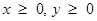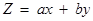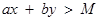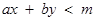1. /
2. CBSE
3. /
4. Class 12
5. /
6. Linear Programming Class 12...

# Linear Programming Class 12 Notes Mathematics### myCBSEguide App

Download the app to get CBSE Sample Papers 2023-24, NCERT Solutions (Revised), Most Important Questions, Previous Year Question Bank, Mock Tests, and Detailed Notes.

Linear Programming class 12 Notes Mathematics in PDF are available for free download in myCBSEguide mobile app. The best app for CBSE students now provides Linear Programming class 12 Notes latest chapter wise notes for quick preparation of CBSE board exams and school-based annual examinations. Class 12 Mathematics notes on chapter 12 Linear Programming are also available for download in CBSE Guide website.

## Linear Programming Class 12 Notes Mathematics

Download CBSE class 12th revision notes for chapter 12 Linear Programming in PDF format for free. Download revision notes for Linear Programming class 12 Notes and score high in exams. These are the Linear Programming class 12 Notes prepared by team of expert teachers. The revision notes help you revise the whole chapter 12 in minutes. Revision notes in exam days is one of the best tips recommended by teachers during exam days.

## CBSE Class 12 Mathematics Revision Notes Chapter 12 Linear Programming

Linear Programming Problem: A linear programming problem is one that is concerned with finding the optimal value (maximum or minimum) of a linear function of several variables (called objective function) subject to the conditions that the variables are non-negative and satisfy a set of linear inequalities (called linear constraints). Variables are sometimes called decision variables and are non-negative.

• A few important linear programming problems are:

(i)       Diet problems

(ii)      Manufacturing problems

(iii)     Transportation problems

(iv)     Allocation problems

• The common region determined by all the constraints including the non-negative constraintsof a linear programming problem is called the feasible region (or solution region) for the problem.
• Points within and on the boundary of the feasible region represent feasible solutions of the constraints. Any point outside the feasible region is an infeasible solution.
• Any point in the feasible region that gives the optimal value (maximum or minimum) of the objective function is called an optimal solution.
• The following Theorems are fundamental in solving linear programming problems:

Theorem 1 Let R be the feasible region (convex polygon) for a linear programming problem and letbe the objective function. When Z has an optimal value (maximum or minimum), where the variables x and y are subject to constraints described by linear inequalities, this optimal value must occur at a corner point (vertex) of the feasible region.

Theorem 2 Let R be the feasible region for a linear programming problem, and let be the objective function.  If R is bounded, then the objective function Z has both a maximum and a minimum value on R and each of these occurs at a corner point (vertex) of R.

• If the feasible region is unbounded, then a maximum or a minimum may not exist. However, if it exists, it must occur at a corner point of R.
• Corner point method : For solving a linear programming problem. The method comprises of the following steps:

(i)     Find the feasible region of the linear programming problem and determine its corner points (vertices).

(ii)   Evaluate the objective functionat each corner point. Let M and m respectively be the largest and smallest values at these points.

(iii)     If the feasible region is bounded, M and m respectively are the maximum and minimum values of the objective function.

• If the feasible region is unbounded, then,

(i)   M is the maximum value of the objective function, if the open half plane determined byhas no point in common with the feasible region. Otherwise, the objective function has no maximum value.

(ii)   m is the minimum value of the objective function, if the open half plane determined byhas no point in common with the feasible region. Otherwise, the objective function has no minimum value.

• If two corner points of the feasible region are both optimal solutions of the same type, i.e., both produce the same maximum or minimum, then any point on the line segment joining these two points is also an optimal solution of the same type.

## CBSE Class 12 Revision Notes and Key Points

Linear Programming class 12 Notes Mathematics. CBSE quick revision note for class-12 Chemistry Physics Math’s, Biology and other subject are very helpful to revise the whole syllabus during exam days. The revision notes covers all important formulas and concepts given in the chapter. Even if you wish to have an overview of a chapter, quick revision notes are here to do if for you. These notes will certainly save your time during stressful exam days.

To download Linear Programming class 12 Notes Mathematics, sample paper for class 12 Physics, Chemistry, Biology, History, Political Science, Economics, Geography, Computer Science, Home Science, Accountancy, Business Studies and Home Science; do check myCBSEguide app or website. myCBSEguide provides sample papers with solution, test papers for chapter-wise practice, NCERT Linear Programming, NCERT Exemplar Linear Programming, quick revision notes for ready reference, CBSE guess papers and CBSE important question papers. Sample Paper all are made available through the best app for CBSE students and myCBSEguide website.### Test Generator

Create question paper PDF and online tests with your own name & logo in minutes.### myCBSEguide

Question Bank, Mock Tests, Exam Papers, NCERT Solutions, Sample Papers, Notes